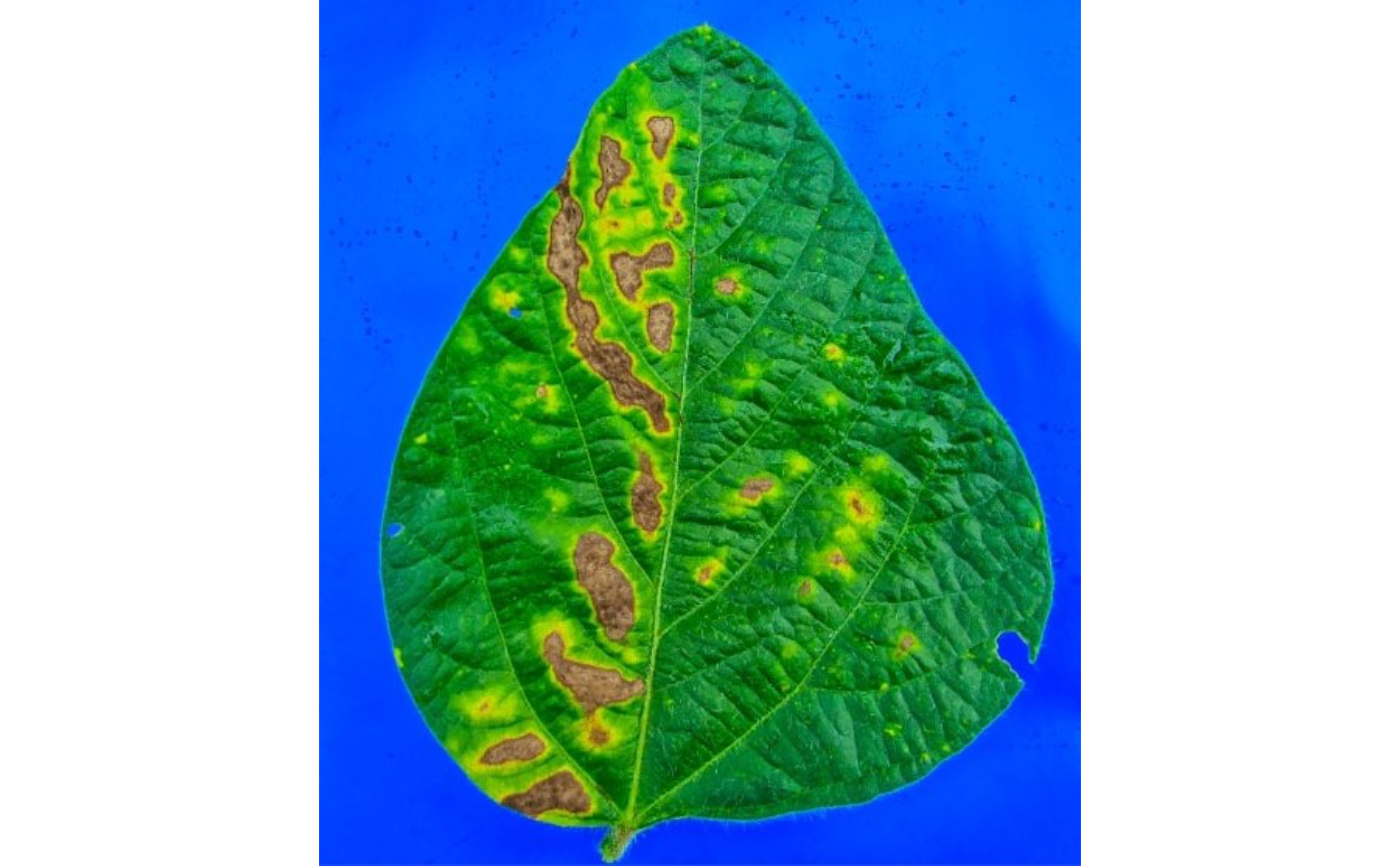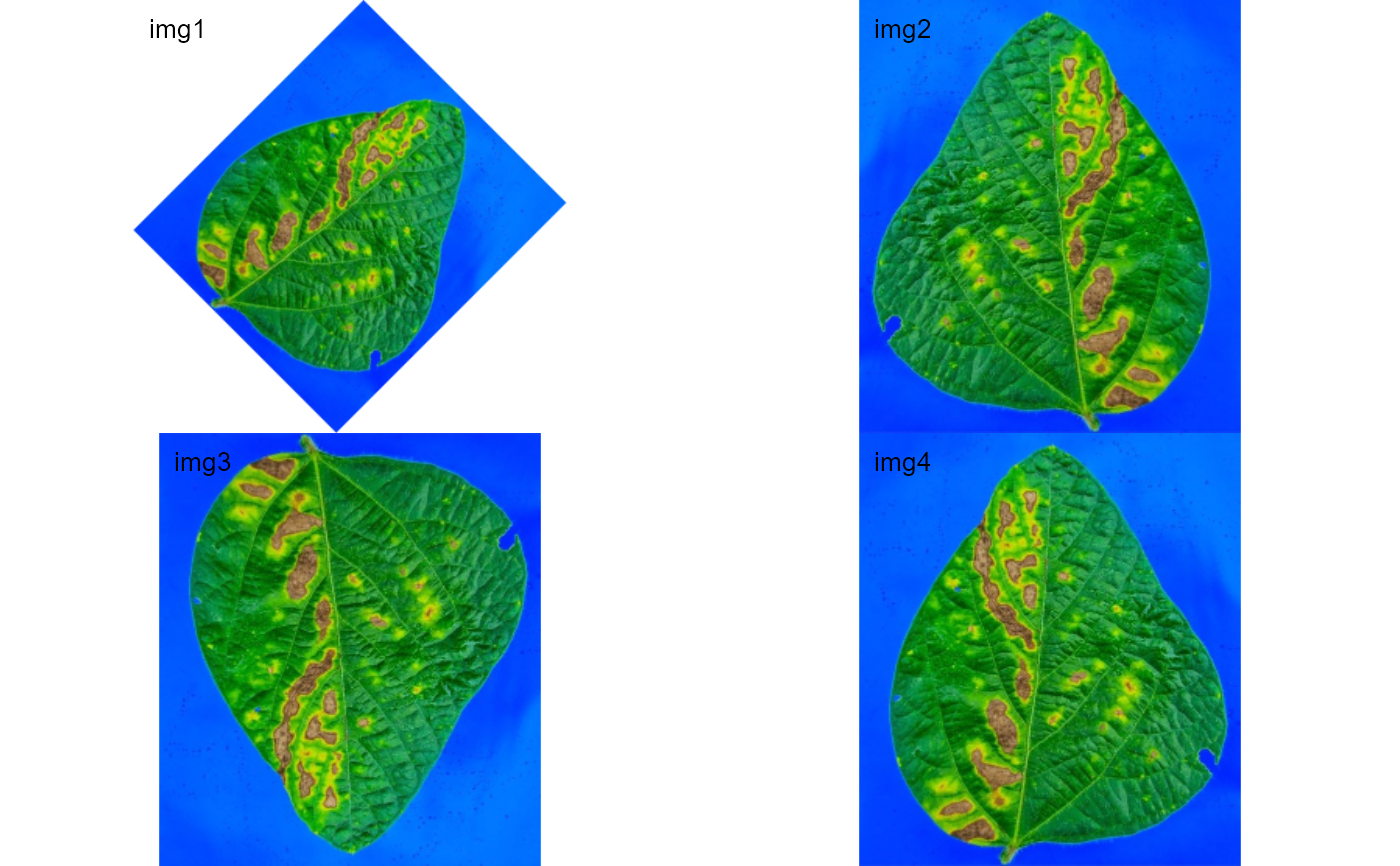Performs image rotation and reflection

• image autocrop() Crops automatically an image to the area of objects.

• image_crop() Crops an image to the desired area.

• image_trim() Remove pixels from the edges of an image (20 by default).

• image_dimension() Gives the dimension (width and height) of an image.

• image_rotate() Rotates the image clockwise by the given angle.

• image_horizontal() Converts (if needed) an image to a horizontal image.

• image_vertical() Converts (if needed) an image to a vertical image.

• image_hreflect() Performs horizontal reflection of the image.

• image_vreflect() Performs vertical reflection of the image.

• image_resize() Resize the image. See more at EBImage::resize().

• image_contrast() Improve contrast locally by performing adaptive histogram equalization. See more at EBImage::clahe().

• image_dilate() Performs image dilatation. See more at EBImage::dilate().

• image_erode() Performs image erosion. See more at EBImage::erode().

• image_opening() Performs an erosion followed by a dilation. See more at EBImage::opening().

• image_closing() Performs a dilation followed by an erosion. See more at EBImage::closing().

• image_filter() Performs median filtering in constant time. See more at EBImage::medianFilter().

• image_blur() Performs blurring filter of images. See more at EBImage::gblur().

• image_skeleton() Performs image skeletonization.

## Usage

image_autocrop(
img,
index = "NB",
edge = 5,
filter = 3,
parallel = FALSE,
workers = NULL,
verbose = TRUE,
plot = FALSE
)

image_crop(
img,
width = NULL,
height = NULL,
viewer = get_pliman_viewer(),
show = "rgb",
parallel = FALSE,
workers = NULL,
verbose = TRUE,
plot = FALSE
)

image_dimension(img, parallel = FALSE, workers = NULL, verbose = TRUE)

image_rotate(
img,
angle,
bg_col = "white",
parallel = FALSE,
workers = NULL,
verbose = TRUE,
plot = TRUE
)

image_horizontal(
img,
parallel = FALSE,
workers = NULL,
verbose = TRUE,
plot = FALSE
)

image_vertical(
img,
parallel = FALSE,
workers = NULL,
verbose = TRUE,
plot = FALSE
)

image_hreflect(
img,
parallel = FALSE,
workers = NULL,
verbose = TRUE,
plot = FALSE
)

image_vreflect(
img,
parallel = FALSE,
workers = NULL,
verbose = TRUE,
plot = FALSE
)

image_resize(
img,
rel_size = 100,
width,
height,
parallel = FALSE,
workers = NULL,
verbose = TRUE,
plot = FALSE
)

image_trim(
img,
edge = NULL,
top = NULL,
bottom = NULL,
left = NULL,
right = NULL,
parallel = FALSE,
workers = NULL,
verbose = TRUE,
plot = FALSE
)

image_dilate(
img,
kern = NULL,
size = NULL,
shape = "disc",
parallel = FALSE,
workers = NULL,
verbose = TRUE,
plot = FALSE
)

image_erode(
img,
kern = NULL,
size = NULL,
shape = "disc",
parallel = FALSE,
workers = NULL,
verbose = TRUE,
plot = FALSE
)

image_opening(
img,
kern = NULL,
size = NULL,
shape = "disc",
parallel = FALSE,
workers = NULL,
verbose = TRUE,
plot = FALSE
)

image_closing(
img,
kern = NULL,
size = NULL,
shape = "disc",
parallel = FALSE,
workers = NULL,
verbose = TRUE,
plot = FALSE
)

image_skeleton(
img,
kern = NULL,
parallel = FALSE,
workers = NULL,
verbose = TRUE,
plot = FALSE,
...
)

image_thinning(
img,
niter = 3,
parallel = FALSE,
workers = NULL,
verbose = TRUE,
plot = FALSE,
...
)

image_filter(
img,
size = 2,
cache = 512,
parallel = FALSE,
workers = NULL,
verbose = TRUE,
plot = FALSE
)

image_blur(
img,
sigma = 3,
parallel = FALSE,
workers = NULL,
verbose = TRUE,
plot = FALSE
)

image_contrast(
img,
parallel = FALSE,
workers = NULL,
verbose = TRUE,
plot = FALSE
)

## Arguments

img

An image or a list of images of class Image.

index

The index to segment the image. See image_index() for more details. Defaults to "NB" (normalized blue).

edge
• for image_autocrop() the number of pixels in the edge of the cropped image. If edge = 0 the image will be cropped to create a bounding rectangle (x and y coordinates) around the image objects.

• for image_trim(), the number of pixels removed from the edges. By default, 20 pixels are removed from all the edges.

filter

Performs median filtering in the binary image. This is useful to remove noise (like dust) and improve the image autocropping method. See more at image_filter(). Set to FALSE to remove the median filtering.

parallel

Processes the images asynchronously (in parallel) in separate R sessions running in the background on the same machine. It may speed up the processing time when image is a list. The number of sections is set up to 70% of available cores.

workers

A positive numeric scalar or a function specifying the maximum number of parallel processes that can be active at the same time.

verbose

If TRUE (default) a summary is shown in the console.

plot

If TRUE plots the modified image. Defaults to FALSE.

width, height
• For image_resize() the Width and height of the resized image. These arguments can be missing. In this case, the image is resized according to the relative size informed in rel_size.

• For image_crop() a numeric vector indicating the pixel range (x and y, respectively) that will be maintained in the cropped image, e.g., width = 100:200

viewer

The viewer option. If not provided, the value is retrieved using get_pliman_viewer(). This option controls the type of viewer to use for interactive plotting. The available options are "base" and "mapview". If set to "base", the base R graphics system is used for interactive plotting. If set to "mapview", the mapview package is used. To set this argument globally for all functions in the package, you can use the set_pliman_viewer() function. For example, you can run set_pliman_viewer("mapview") to set the viewer option to "mapview" for all functions.

show

How to plot in mapview viewer, either "rgb" or "index".

angle

The rotation angle in degrees.

bg_col

Color used to fill the background pixels, defaults to "white".

rel_size

The relative size of the resized image. Defaults to 100. For example, setting rel_size = 50 to an image of width 1280 x 720, the new image will have a size of 640 x 360.

top, bottom, left, right

The number of pixels removed from top, bottom, left, and right when using image_trim().

kern

An Image object or an array, containing the structuring element. Defaults to a brushe generated with EBImage::makeBrush().

size
• For image_filter() is the median filter radius (integer). Defaults to 3.

• For image_dilate() and image_erode() is an odd number containing the size of the brush in pixels. Even numbers are rounded to the next odd one. The default depends on the image resolution and is computed as the image resolution (megapixels) times 20.

shape

A character vector indicating the shape of the brush. Can be box, disc, diamond, Gaussian or line. Default is disc.

...

Additional arguments passed on to image_binary().

niter

The number of iterations to perform in the thinning procedure. Defaults to 3. Set to NULL to iterate until the binary image is no longer changing.

cache

The the L2 cache size of the system CPU in kB (integer). Defaults to 512.

sigma

A numeric denoting the standard deviation of the Gaussian filter used for blurring. Defaults to 3.

## Value

• image_skeleton() returns a binary Image object.

• All other functions returns a modified version of image depending on the image_*() function used.

• If image is a list, a list of the same length will be returned.

## Author

Tiago Olivoto tiagoolivoto@gmail.com

## Examples

library(pliman)
img <- image_pliman("sev_leaf.jpg")
plot(img)img <- image_resize(img, 50)
img1 <- image_rotate(img, 45)
img2 <- image_hreflect(img)
img3 <- image_vreflect(img)
img4 <- image_vertical(img)
image_combine(img1, img2, img3, img4)